### Contest Math I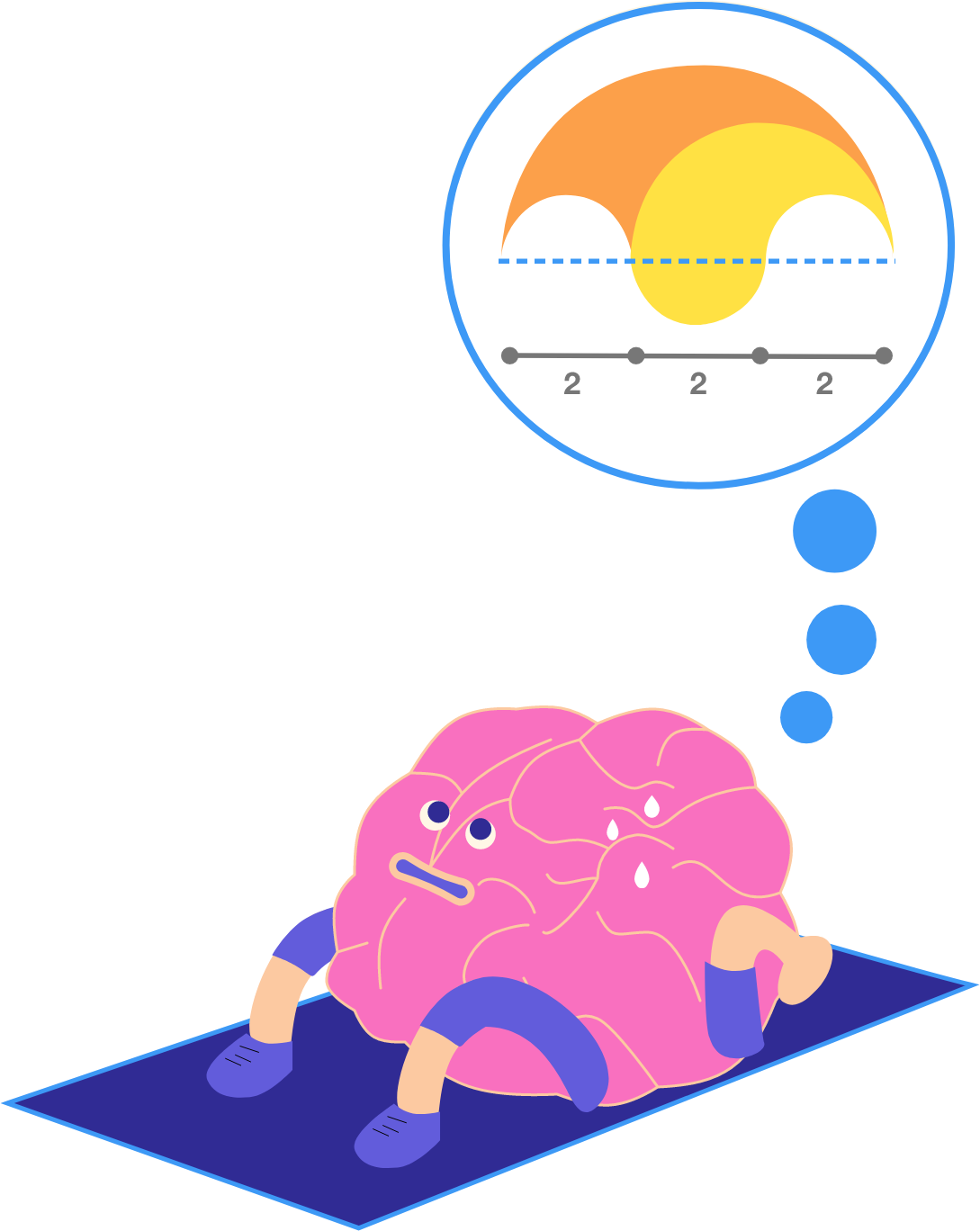In this quiz, you'll solve five problems that require both efficiency and creativity. Each problem can be solved in several different ways, and none of them require a 'brute-force' approach. Instead, try to be efficient in your problem solving and check out the solutions for each problem to learn the strategies you don't already know.

# Math Requires Creativity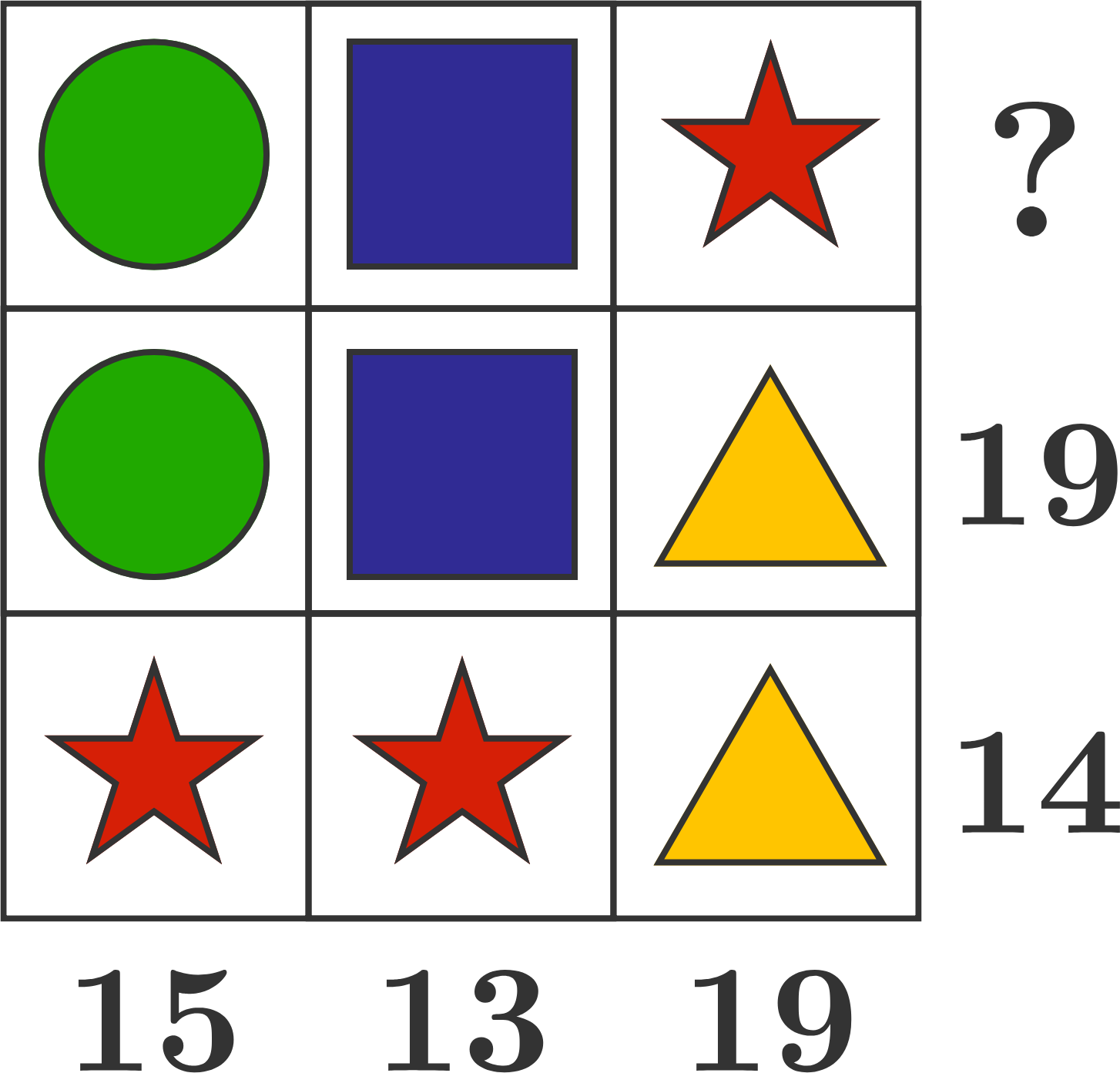In this array puzzle, each shape has a specific value. The number next to each row or column represents the sum of the values in that row or column.

What number should replace the question mark?

# Math Requires Creativity

Do you feel like you solved the previous array problem efficiently?

If not, consider going back and checking out the problem's three solutions.Being efficient when problem solving doesn't just help you with your speed during competitions. It's also an important part of all advanced and professional mathematics to be able to recognize simpler and more efficient strategies for solving problems. That's why, for each problem in this quiz, we've written up multiple different solutions, all of which are creative, efficient ways to solve the problem.

# Math Requires Creativity

Here is another problem that doesn't require a calculator or brute force approach. You can solve it efficiently by figuring out how to compare the two numbers without actually calculating the exact value of either.

Which is larger,

$\Large {3}^{12} \quad \text{or} \quad {5}^{9}\, ?$

# Math Requires Creativity

If Jasmine flips a fair coin $\textbf6$ times in a row, what is the probability that she gets more heads than tails?# Math Requires Creativity

Now we'll wrap up with two geometry problems. Neither of these problems is about knowing "the right formula or equation." Instead, use your own logic and intuition!

The hexagon below is regular. Which of the two colored areas is larger?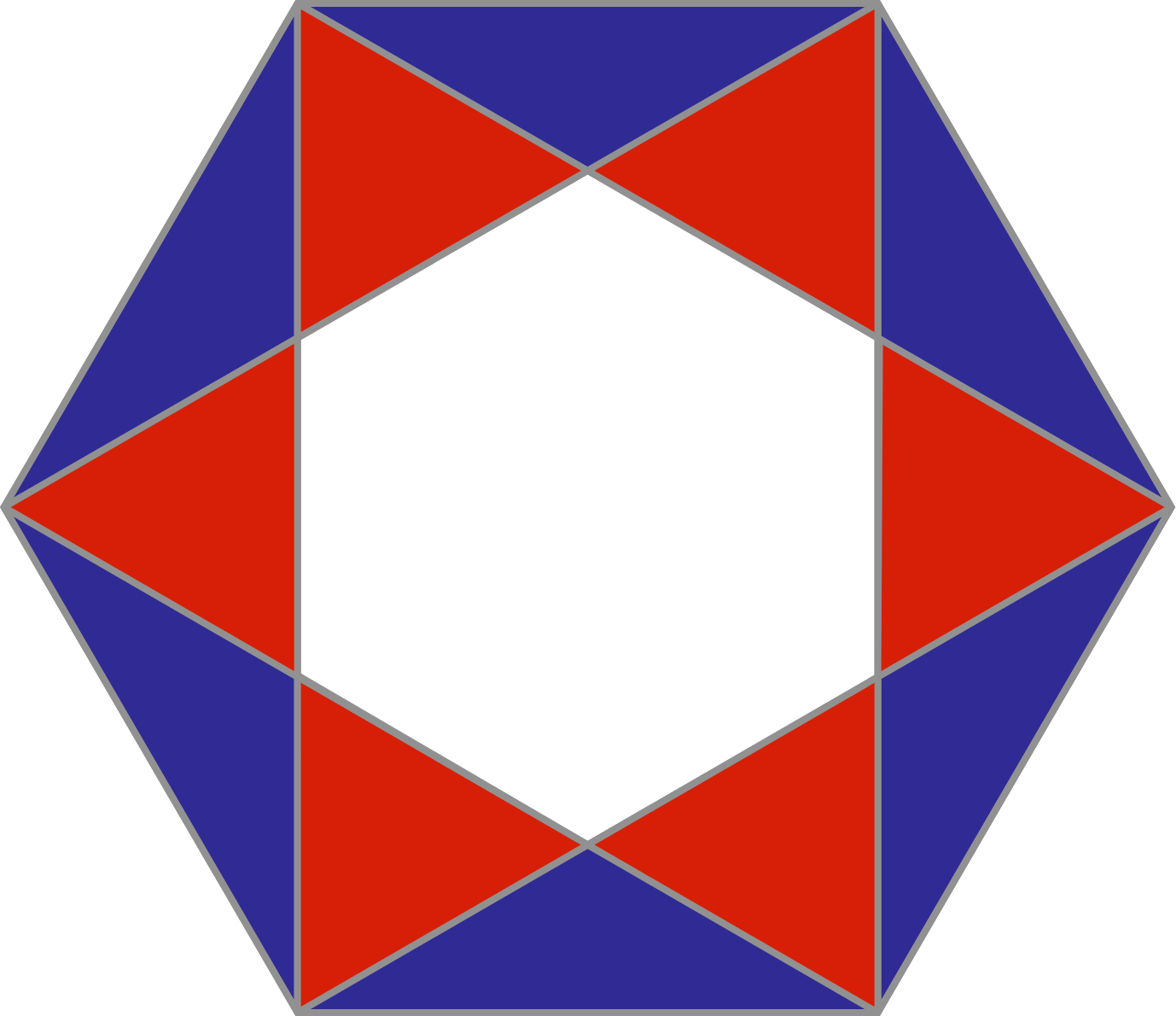# Math Requires Creativity

What is the sum of the red angles?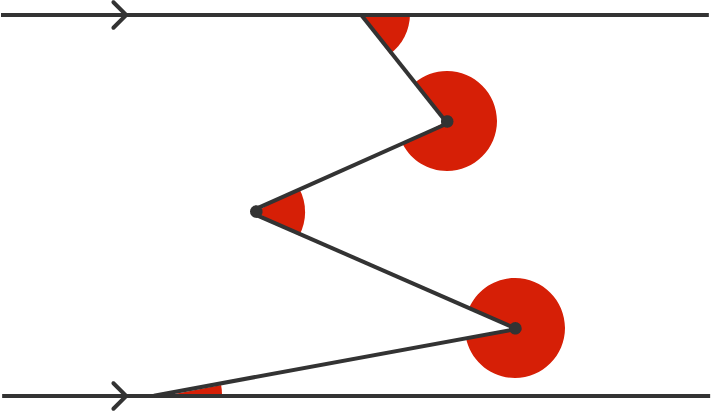# Math Requires Creativity

Is speed all that matters?

No. Math contests are competitive tests of your speed and accuracy executing the mathematical techniques you've learned in classes, but you'll find that most competitions also require problem-solving strategy and creativity beyond the standard strategies typically taught in a classroom.

In this course, we'll introduce you to the kinds of challenges you can expect in competitions at the level of the AMC8 and MATHCOUNTS. We've grouped the curriculum into four categories so that you can decide the order and intensity of your training in each area yourself.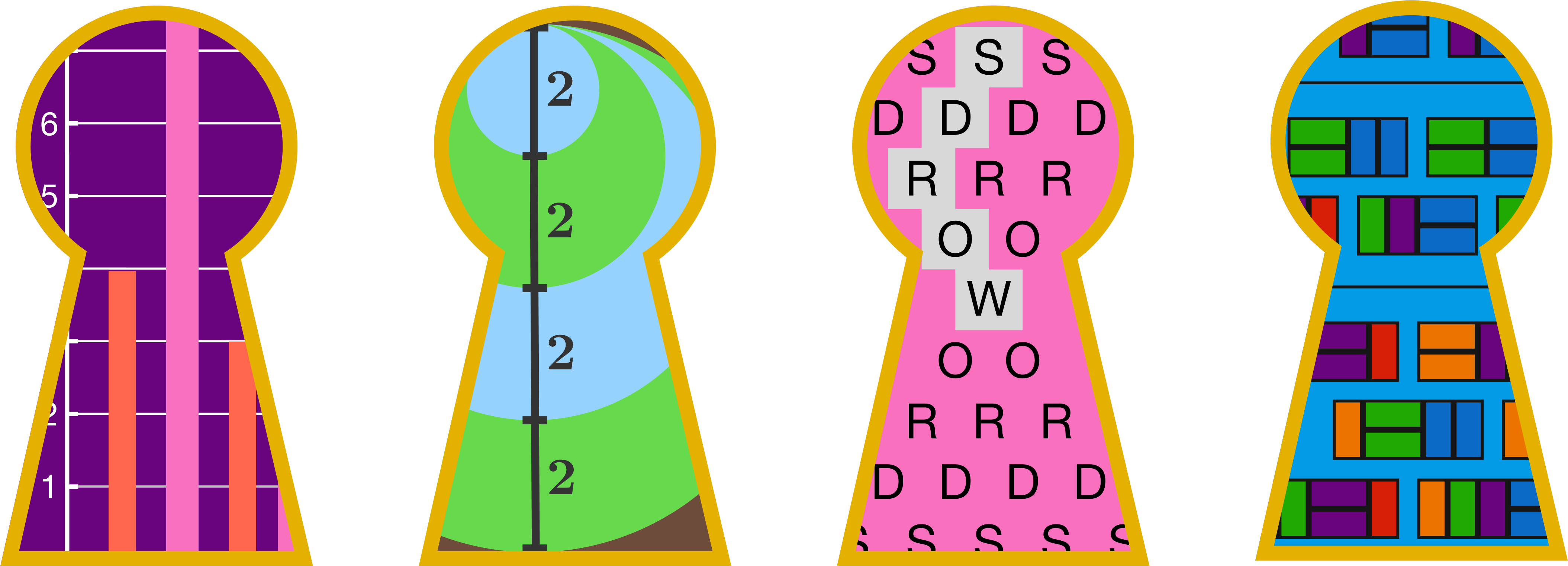The four categories and the nine corresponding chapters in this course are as follows:

• Algebra (Equations and Data)
• Geometry (Measures, Similarity, and Composites)
• Combinatorics (Counting and Probability)
• Number Theory (Efficiency and Factorization)

×